Open in App
Not now

# Class 8 RD Sharma Solutions – Chapter 16 Understanding Shapes Quadrilaterals – Exercise 16.1 | Set 1

• Last Updated : 08 Apr, 2021

### Question 1. Define the following terms:

Solution:

(i) Quadrilateral: Let’s A, B, C and D are four points in a plane such that :

(a) no three of them are collinear.

(b) The line segments AB, BC, CD and DA do not intersect except at their end points. Then an Enclosed figure with four sides is termed as Quadrilateral.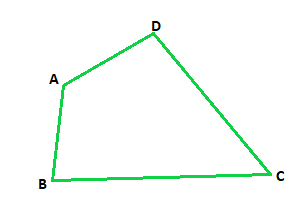(ii) Convex Quadrilateral: If the line containing any side of the quadrilateral has the remaining vertices on the same side of it then it is known as Convex                            Quadrilateral.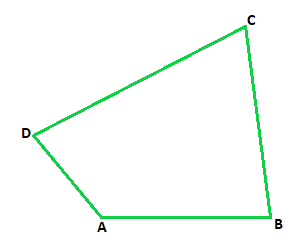In the above figure vertices A, B lie on the same side of line CD, vertices B, C lie on the same side of line DA, vertices C, D lie on the same side of line AB, vertices D, A lie on the same side of line BC.

### Question 2. In a quadrilateral, define each of the following:

(i) Sides

(ii) Vertices

(iii) Angles

(iv) Diagonals

(vii) Opposite sides

(viii) Opposite angles

(ix) Interior

(x) Exterior

Solution: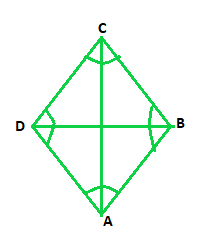(i) Sides: In a quadrilateral all the sides may have same or of different length. The four line segments AB, BC, CD and DA are called as sides of quadrilateral.

(ii) Vertices: Vertices are the angular points where two edges or sides of quadrilateral meet. A, B, C and D are the four vertices in a quadrilateral.

(iii) Angles: Angle is the inclination between two sides of a quadrilateral. i.e. meeting point of two sides is an angle. ABC, BCA, CDA and DAB are the four angles in a quadrilateral.

(iv) Diagonals: The lines joining two opposite vertices is called the diagonals in a quadrilateral. BD and AC are the two diagonals.

(v) Adjacent angles: Angles having one common arm onto the sides is called the adjacent angles. ABC, BCD are adjacent angles in a quadrilateral.

(vi) Adjacent sides: When two sides have common endpoint is termed as adjacent sides. AB BC, BC CA, CD DA, DA AB are pairs of adjacent sides in a quadrilateral.

(vii) Opposite sides: Opposite sides when they don’t meet at any point is termed as opposite sides. AB CD, BC DA are the pairs of opposite sides in a quadrilateral.

(viii) Opposite angles: Two angles, which are not adjacent angles are termed as opposite angles. A and C, angles B and D are opposite angles in a quadrilateral.

(ix) Interior: The part of plane when points are enclosed within the quadrilateral is called as interior.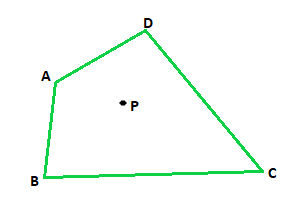(x) Exterior: The part of plane when points are not enclosed within the quadrilateral is called as exterior.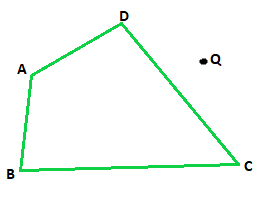### Question 3. Complete each of the following, so as to make a true statement :

(i) A quadrilateral has ________ sides.

(iii) A quadrilateral has ________, no three of which are ________.

(v) The number of pairs of adjacent angles of a quadrilateral is ________.

(vi) The number of pairs of opposite angles of a quadrilateral is ________.

(vii) The sum of the angles of a quadrilateral is ________.

(viii) A diagonal of a quadrilateral is a line segment that joins two ________ vertices of the quadrilateral.

(ix) The sum of the angles of a quadrilateral is ________ right angles.

(x) The measure of each angle of a convex quadrilateral is ________ 180°.

(xi) In a quadrilateral the point of intersection of the diagonals lies in ________ of the quadrilateral.

(xii) A point is in the interior of a convex quadrilateral, if it is in the ________ of its two opposite angles.

(xiii) A quadrilateral is convex if for each side, the remaining ________ lie on the same side of the line containing the side.

Solution:

(i) A quadrilateral has four sides.

(ii) A quadrilateral has four angles.

(iii) A quadrilateral has four, no three of which are collinear.

(iv) A quadrilateral has two diagonals.

(v) The number of pairs of adjacent angles of a quadrilateral is four.

(vi) The number of pairs of opposite angles of a quadrilateral is two.

(vii) The sum of the angles of a quadrilateral is 3600.

(viii) A diagonal of a quadrilateral is a line segment that joins two opposite vertices of the quadrilateral.

(ix) The sum of the angles of a quadrilateral is four right angles.

(x) The measure of each angle of a convex quadrilateral is less than 180°.

(xi) In a quadrilateral the point of intersection of the diagonals lies in interior of the quadrilateral.

(xii) A point is in the interior of a convex quadrilateral, if it is in the interiors of its two opposite angles.

(xiii) A quadrilateral is convex if for each side, the remaining vertices lie on the same side of the line containing the side.

### Question 4. In Fig., ABCD is a quadrilateral.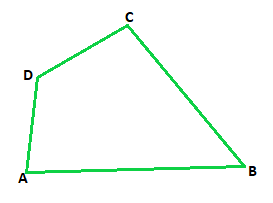(i) Name a pair of adjacent sides.

(ii) Name a pair of opposite sides.

(iii) How many pairs of adjacent sides are there?

(iv) How many pairs of opposite sides are there?

(v) Name a pair of adjacent angles.

(vi) Name a pair of opposite angles.

(vii) How many pairs of adjacent angles are there?

(viii) How many pairs of opposite angles are there?

Solution:

(i) Adjacent sides are : AB, BC or BC, CD or CD, DA or AD, AB

(ii) Opposite sides are : AB, CD or BC, DA

(iii) Four pairs of adjacent sides are : AB BC, BC CD, CD DA and DA AB

(iv) Two pairs of opposite sides are :  AB, DC and DA, BC

(v) Four pairs of Adjacent angles are : D∠AB A∠BC, A∠BC B∠CA, B∠CA C∠DA or C∠DA D∠AB

(vi) Four pair of opposite angles are: D∠AB B∠CA and A∠BC C∠DA

(vii) Four pairs of adjacent angles are : D∠AB A∠BC, A∠BC B∠CA, B∠CA C∠DA and C∠DA D∠AB

(viii) Two pairs of opposite angles are : D∠AB B∠CA and A∠BC C∠DA

### Question 5. The angles of a quadrilateral are 110°, 72°, 55°, and x°. Find the value of x.

Solution:

As we know that Sum of angles of a quadrilateral is = 360°

Therefore,

110° + 72° + 55° + x° = 360°

x° = 360° – 237°

x° = 123o

Hence, Value of x is 123o

### Question 6. The three angles of a quadrilateral are respectively equal to 110°, 50° and 40°. Find its fourth angle.

Solution:

As we know that Sum of angles of a quadrilateral is = 360°

Therefore,

110° + 50° + 40° + x° = 360°

x° = 360° – 200°

x° = 160o

Hence, Value of fourth angle is 160o

### Question 7. A quadrilateral has three acute angles each measures 80°. What is the measure of the fourth angle?

Solution:

As we know that Sum of angles of a quadrilateral is = 360°

Therefore,

80° + 80° + 80° + x° = 360°

x° = 360° – 240°

x° = 120o

Hence, Value of fourth angle is 120o

### Question 8. A quadrilateral has all its four angles of the same measure. What is the measure of each?

Solution:

As we know that Sum of angles of a quadrilateral is = 360°

Let us assume that each angle be xo

Therefore,

xo + xo + xo + xo = 360o

xo = 360o/4 = 90o

Hence, Value of angle is 90o each.

### Question 9. Two angles of a quadrilateral are of measure 65° and the other two angles are equal. What is the measure of each of these two angles?

Solution:

As we know that Sum of angles of a quadrilateral is = 360°

Let us assume that each angle be xo

Therefore,

65o + 65o + xo + xo = 360o

2xo = 360o – 130o

xo = 230o/2 = 115o

Hence, Value of two angles is 115o each.

### Question 10. Three angles of a quadrilateral are equal. Fourth angle is of measure 150°. What is the measure of equal angles?

Solution:

As we know that Sum of angles of a quadrilateral is = 360°

Let us assume that each angle be xo

Therefore,

150o + xo + xo + xo = 360o

3xo = 360o – 150o

xo = 210o/3 = 70o

Hence, Value of equal angles is 70o each.

### Question 11. The four angles of a quadrilateral are as 3:5:7:9. Find the angles.

Solution:

As we know that Sum of angles of a quadrilateral is = 360°

Let us assume that each angle be xo

Therefore,

3xo + 5xo + 7xo + 9xo = 360o

24xo = 360o

xo = 360o/24 = 15o

Hence, Value of angles are :

3x = 3 × 15 = 45o

5x = 5 × 15 = 75o

7x = 7 × 15 = 105o

9x = 9 × 15 = 135o

Value of angles are 45o, 75o, 105o, 135o

### Question 12. If the sum of the two angles of a quadrilateral is 180°. What is the sum of the remaining two angles?

Solution:

As we know that Sum of angles of a quadrilateral is = 360°

Let us assume that the sum of two angles be 180o

Let’s angle be xo

Therefore,

180o + xo = 360o

xo = 360o – 180o

xo = 180o

Hence, Sum of remaining two angles is 180o

### Chapter 16 Understanding Shapes Quadrilaterals – Exercise 16.1 | Set 2

My Personal Notes arrow_drop_up